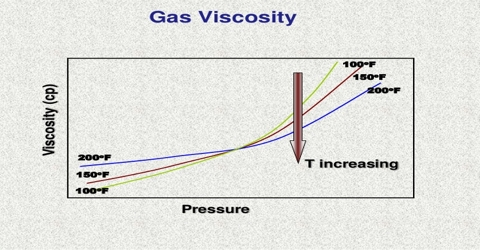# Viscosity of Gasses

Viscosity of Gasses

When a mass of gas is caused to stream of flow in any given direction there is transfer of momentum from one layer of gas to neighboring layer due to random movement of the molecules. This transfer of momentum causes frictional, or dragging force trying to retard the motion of the fast moving layers giving rise to viscosity of gases.

Viscosity or the co-efficient of viscosity is defined as the force per unit area exerted between two parallel layers one cm apart, when the velocity of streaming differs by unity in the two layers.

The mean free path of a gas may be determined from measurements of its viscosity. Viscosity of gas may be measured in the same way as for a liquid. With the help of the kinetic theory a relation between the coefficient of viscosity, η, and the mean free path, l, has been deduced. The relation is:

η = ½ mncl

where m is the mass of one molecule, n is the number of molecules in l ml, c is the average velocity and l is the mean free path.